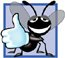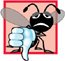C++ enables several functions of the same name to be defined, as long as these functions have different sets of parameters (at least as far as the parameter types or the number of parameters or the order of the parameter types are concerned). This capability is called function overloading. When an overloaded function is called, the C++ compiler selects the proper function by examining the number, types and order of the arguments in the call. Function overloading is commonly used to create several functions of the same name that perform similar tasks, but on different data types. For example, many functions in the math library are overloaded for different numeric data types.

 The C++ standard requires float, double and long double overloaded versions of the math library functions discussed in Section 6.3.

Good Programming Practice 6.8Overloading functions that perform closely related tasks can make programs more readable and understandable.

Figure 6.24 uses overloaded square functions to calculate the square of an int (lines 812) and the square of a double (lines 1519). Line 23 invokes the int version of function square by passing the literal value 7. C++ treats whole number literal values as type int by default. Similarly, line 25 invokes the double version of function square by passing the literal value 7.5, which C++ treats as a double value by default. In each case the compiler chooses the proper function to call, based on the type of the argument. The last two lines of the output window confirm that the proper function was called in each case.

Figure 6.24. Overloaded square functions.

(This item is displayed on pages 283 - 284 in the print version)

1 // Fig. 6.24: fig06_24.cpp
2 // Overloaded functions.
3 #include
4 using std::cout;
5 using std::endl;
6
7 // function square for int values
8 int square( int x )
9 {
10  cout << "square of integer " << x << " is ";
11  return x * x;
12 } // end function square with int argument
13
14 // function square for double values
15 double square( double y )
16 {
17  cout << "square of double " << y << " is ";
18  return y * y;
19 } // end function square with double argument
20
21 int main()
22 {
23 cout << square( 7 ); // calls int version
24 cout << endl;
25 cout << square( 7.5 ); // calls double version
26 cout << endl;
27 return 0; // indicates successful termination
28 } // end main

 square of integer 7 is 49 square of double 7.5 is 56.25

How the Compiler Differentiates Overloaded Functions

Overloaded functions are distinguished by their signatures. A signature is a combination of a function's name and its parameter types (in order). The compiler encodes each function identifier with the number and types of its parameters (sometimes referred to as name mangling or name decoration) to enable type-safe linkage. Type-safe linkage ensures that the proper overloaded function is called and that the types of the arguments conform to the types of the parameters.

Figure 6.25 was compiled with the Borland C++ 5.6.4 command-line compiler. Rather than showing the execution output of the program (as we normally would), we show the mangled function names produced in assembly language by Borland C++. Each mangled name begins with @ followed by the function name. The function name is then separated from the mangled parameter list by \$q. In the parameter list for function nothing2 (line 25; see the fourth output line), c represents a char, i represents an int, rf represents a float & (i.e., a reference to a float) and rd represents a double & (i.e., a reference to a double). In the parameter list for function nothing1, i represents an int, f represents a float, c represents a char and ri represents an int &. The two square functions are distinguished by their parameter lists; one specifies d for double and the other specifies i for int. The return types of the functions are not specified in the mangled names. Overloaded functions can have different return types, but if they do, they must also have different parameter lists. Again, you cannot have two functions with the same signature and different return types. Note that function name mangling is compiler specific. Also note that function main is not mangled, because it cannot be overloaded.

Figure 6.25. Name mangling to enable type-safe linkage.

1 // Fig. 6.25: fig06_25.cpp
2 // Name mangling.
3
4 // function square for int values
5 int square( int x )
6 {
7 return x * x;
8 } // end function square
9
10 // function square for double values
11 double square( double y )
12 {
13 return y * y;
14 } // end function square
15
16 // function that receives arguments of types
17 // int, float, char and int &
18 void nothing1( int a, float b, char c, int &d )
19 {
20 // empty function body
21 } // end function nothing1
22
23 // function that receives arguments of types
24 // char, int, float & and double &
25 int nothing2( char a, int b, float &c, double &d )
26 {
27 return 0;
28 } // end function nothing2
29
30 int main()
31 {
32 return 0; // indicates successful termination
33 } // end main

 @square\$qi @square\$qd @nothing1\$qifcri @nothing2\$qcirfrd _main

Common Programming Error 6.21Creating overloaded functions with identical parameter lists and different return types is a compilation error.

The compiler uses only the parameter lists to distinguish between functions of the same name. Overloaded functions need not have the same number of parameters. Programmers should use caution when overloading functions with default parameters, because this may cause ambiguity.

Common Programming Error 6.22A function with default arguments omitted might be called identically to another overloaded function; this is a compilation error. For example, having in a program both a function that explicitly takes no arguments and a function of the same name that contains all default arguments results in a compilation error when an attempt is made to use that function name in a call passing no arguments. The compiler does not know which version of the function to choose.

In Chapter 11, we discuss how to overload operators to define how they should operate on objects of user-defined data types. (In fact, we have been using many overloaded operators to this point, including the stream insertion operator << and the stream extraction operator >>, each of which is overloaded to be able to display data of all the fundamental types. We say more about overloading << and >> to be able to handle objects of user-defined types in Chapter 11.) Section 6.18 introduces function templates for automatically generating overloaded functions that perform identical tasks on different data types.C++ How to Program (5th Edition)
ISBN: 0131857576
EAN: 2147483647
Year: 2004
Pages: 627

Similar book on Amazon